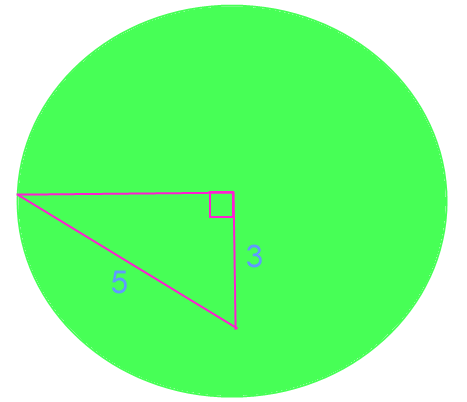# Geometry with Vanilla JavaScript

In this lesson, we will solve Geometry problems using JavaScript. On the bottom of the page you will find a code editor pre-loaded with the example problems followed by problems for you to try on your own!

## Problem 1: Area

Susie Smith owns a landcare service. She charges \$0.02 per square foot. Elliot's yard is 100 feet by 150 feet. How much will Susie charge Elliot?``````let length = 100; // length
let width = 150; // width
let area = 100 * 150;  // length * width
let price = .02 * area;

// format to USD
const formatter = new Intl.NumberFormat('en-US', {
style: 'currency',
currency: 'USD',
minimumFractionDigits: 2
})

// print amount
console.log(formatter.format(price));
``````

## Problem 2: Pythagorean Theorem

What is the length of the length of the hypotenuse in the diagra below?``````// Problem 2: Pythagorean theorem
// a**2 + b**2= c**
a = 12;
let b =8;
let c = Math.sqrt((a ** 2) + (b ** 2));
console.log("Problem 2: " + c);``````

## Problem 3: Supplimentry Angles

Angles a and b are supplimentry. Angle a is 120o. How many degrees is angle b?``````// Problem 3: Supplmentry Angles
// suplimentry angles equal 180 degrees
let angle_a = 120;
let angle_b = 180 - angle_a;
console.log("Problem 3: Angle b is " + angle_b + " degrees");``````

## Problem 4: Perimeter

Suzie Smith has been hired to place a fence around the perimeter of Carlos's yard. His yard is rectangular. It is 20 yards wide. It is 20% longer than it is wide. Suzie charges \$15 per yard of fencing. How much will Carlos pay?

``````let yard_length = 20;
let yard_width = 1.2 * 20;
let perimeter = (2 * yard_length) + (2 * yard_width);
let cost = 15 * perimeter;

// format to USD
const formatter = new Intl.NumberFormat('en-US', {
style: 'currency',
currency: 'USD',
minimumFractionDigits: 2
});

console.log("Problem 4: " + formatter.format(cost));``````

## Problem 5: Circumfrence

Shana has a circular yard and has hired Suzie Smith to put a fence around it. This is a custom job, so Suzie will charge \$22 per yard of fencing. If the diameter of Shana's yard is 15 yards, how much will the fence cost?

``````// Problem 5: Circumference
// Circumference = π × diameter
let diameter = 15;
let circumference = Math.PI * 15;
let custom_cost = 22 * circumference;

console.log("Problem 5: " + formatter.format(custom_cost));``````

## Problem 6:

Diedra also has a circular yard and wants Suzie Smith to put up a fence. The custom cost per yard will also be \$22. Diedra has a garden inside her yard that is right-triangle shaped. See the diagram below. One side of the garden is the radius of Diedra's yard. Another side of her garden measures 3 yards. The hypotenuse of her garden measures 5 yards. How much will a custom fence around Diedra's yard cost?``````// Problem 6: Right Triangle in Circle
let side_b = 3;
let hypot = 5;
// radius**2 + b**2 = hypot**2
// radius**2 = hypot**2 - side_b**2
// radius = sqrt(hypot**2 - side_b**2)
let radius = Math.sqrt(hypot**2 - side_b**2);
// circumference = 2 * r *  π
let yard_circumference = 2 * radius * Math.PI;
// circumference * price per yard
let fence_cost = yard_circumference * 22;

console.log("Problem 6: " + formatter.format(fence_cost));``````

## Run the Code

Run the code above in the editor below to observe the output.

## Geometry with JavaScript Practice Problems

Now it's your turn to give it a try! Write JavaScript code to solve the following Geometry problems.

### (1) Area

Mario is buying new carpet for his den. The carpet he picked out costs \$22 per square foot. Mario's den is rectangular. It is 12 feet wide and 20 feet long. How much will the carpet cost Mario?

### (2) Circumference

Ellen has a circular mirror. She wants to put decrotive trim around the circumference of her mirror. If the mirror's radius is 12 inches, how many inches of decortive trim does Ellen need?

### (3) Angles

Line b is a striaght verticle line. Line a intersects line b. Look at the diagram below. Given angle x is equal to 135o, solve for angles y and z.### (4) Right Triangle

A right triangle has 2 sides and a hypotenuse. The first two size measure 4 inches and 6 inches. What is the length of the hypotenuse?